# Precalculus : Solving Polynomial Equations

## Example Questions

2 Next →

### Example Question #6 : Solve A Polynomial By Factoring

Solve this polynomial by factoring if it is factorable: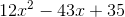Possible Answers:

Not factorable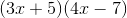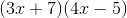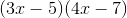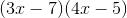Correct answer:Explanation:To factor this polynomial it is prudent to recognize that there will only be two factors since the highest power is.

Then ask what numbers multiply to equal postive 35.

Next, what numbers can multiply to equal positive 12.

Let's try 7 and 5 for the last term and 3 and 4 for the first term.

Be sure to put an "x" in the first term of each factor.

Choose the signs based on what the polynomial calls for. In our case we choose negative signs to get positive 35.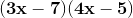Foil these two factors and we get.

2 Next →

### All Precalculus Resources